# Colleges with the highest SAT scores in Illinois

Top 10 colleges in Illinois with the highest SAT scores
Looking for the colleges with the highest SAT scores in Illinois? Well you're in luck! We've compiled a national college database and have created a list of the top 10 universities with the highest SAT scores in Illinois. These are the schools whose applicants had the highest average SAT scores in Illinois. And, since these tests are meant to determine academic prowess, they are arguably the schools with the most academically proficient students. You could even say these are the best colleges in Illinois. We also include each college's ACT scores and acceptance rate so that you can see where you would have the easiest or hardest time getting in. Read on to find out more.

## University of Chicago SAT scores

The average SAT score for University of Chicago is 1520.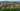The average SAT score of 1520 breaks down into:

• SAT math: 775

The average ACT score for University of Chicago is 34 and their acceptance rate is 7.3%.

## Northwestern University SAT scores

The average SAT score for Northwestern University is 1495.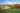The average SAT score of 1495 breaks down into:

• SAT math: 765

The average ACT score for Northwestern University is 34 and their acceptance rate is 9.1%.

## University of Illinois at Urbana-Champaign SAT scores

The average SAT score for University of Illinois at Urbana-Champaign is 1350.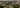The average SAT score of 1350 breaks down into:

• SAT math: 700

The average ACT score for University of Illinois at Urbana-Champaign is 29 and their acceptance rate is 62.2%.

## Illinois Institute of Technology SAT scores

The average SAT score for Illinois Institute of Technology is 1340.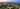The average SAT score of 1340 breaks down into:

• SAT math: 710

The average ACT score for Illinois Institute of Technology is 28 and their acceptance rate is 52.7%.

## Wheaton College SAT scores

The average SAT score for Wheaton College is 1315.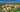The average SAT score of 1315 breaks down into:

• SAT math: 665

The average ACT score for Wheaton College is 29 and their acceptance rate is 82.6%.

## Illinois Wesleyan University SAT scores

The average SAT score for Illinois Wesleyan University is 1310.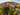The average SAT score of 1310 breaks down into:

• SAT math: 690

The average ACT score for Illinois Wesleyan University is 27 and their acceptance rate is 60.9%.

## Augustana College SAT scores

The average SAT score for Augustana College is 1260.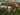The average SAT score of 1260 breaks down into:

• SAT math: 630

The average ACT score for Augustana College is 27 and their acceptance rate is 59%.

## School of the Art Institute of Chicago SAT scores

The average SAT score for School of the Art Institute of Chicago is 1230.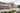The average SAT score of 1230 breaks down into:

• SAT math: 610

The average ACT score for School of the Art Institute of Chicago is 25 and their acceptance rate is 48.6%.

## Knox College SAT scores

The average SAT score for Knox College is 1220.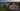The average SAT score of 1220 breaks down into:

• SAT math: 610

The average ACT score for Knox College is 27 and their acceptance rate is 74.2%.

## Lake Forest College SAT scores

The average SAT score for Lake Forest College is 1219.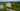The average SAT score of 1219 breaks down into:

• SAT math: 610